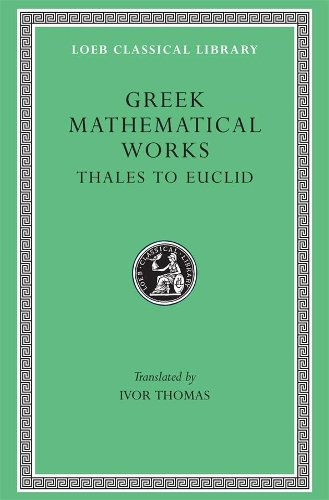•# Greek Mathematical Works: Thales to Euclid Volume I - Loeb Classical Library (Hardback)

(translator)
£19.95
Hardback 576 Pages / Published: 31/01/1939
• In stock

Available

Your local Waterstones may have stock of this item. Please check by using Click & Collect

The wonderful achievement of Greek mathematics is here illustrated in two volumes of selected mathematical works. Volume I contains: The divisions of mathematics; mathematics in Greek education; calculation; arithmetical notation and operations, including square root and cube root; Pythagorean arithmetic, including properties of numbers; square root of 2; proportion and means; algebraic equations; Proclus; Thales; Pythagorean geometry; Democritus; Hippocrates of Chios; duplicating the cube and squaring the circle; trisecting angles; Theaetetus; Plato; Eudoxus of Cnidus (pyramid, cone, etc.); Aristotle (the infinite, the lever); Euclid.

Volume II (Loeb Classical Library no. 362) contains: Aristarchus (distances of sun and moon); Archimedes (cylinder, sphere, cubic equations; conoids; spheroids; spiral; expression of large numbers; mechanics; hydrostatics); Eratosthenes (measurement of the earth); Apollonius (conic sections and other works); later development of geometry; trigonometry (including Ptolemy's table of sines); mensuration: Heron of Alexandria; algebra: Diophantus (determinate and indeterminate equations); the revival of geometry: Pappus.

Publisher: Harvard University Press
ISBN: 9780674993693
Number of pages: 576
Weight: 354 g
Dimensions: 162 x 108 x 31 mm